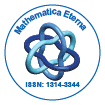# Mathematica EternaOpen Access

ISSN: 1314-3344

## Abstract

### Oscillation of Second Order Nonlinear Neutral Advanced Functional Difference Equations

A. Murugesan and K. Ammamuthu

In this paper, we establish some sufficient conditions for the oscillations of all solutions of the second order nonlinear neutral advanced functional difference equations ∆[r(n)∆(x(n) + p(n)x(n + τ ))] + q(n)f(x(n + σ)) = 0; n ≥ n0, (∗) where P∞ n=n0 1 r(n) = ∞ or P∞ n=n0 1 r(n) < ∞, and 0 ≤ p(n) ≤ p0 < ∞, τ is an integer, and σ is a positive integer. The results proved here improve some known results in the literature.

Top